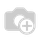# How to get date max in next month?

If we will take date max in this month, can use this code :

date_now = datetime.today()
date_max = date_now.max.day

But how to take date max in next month?You can try following code to get next month's last day:

import datetime

from dateutil.relativedelta import relativedelta

next_month_max_day =((datetime.datetime.today().date() + relativedelta(months=+1,day=31))).dayTry this,

from dateutil.relativedelta import relativedelta
from datetime import datetime
next_month = datetime.today() + relativedelta(months=1)
date_max=next_month.max.dayimport calendar
date_now = datetime.today()
day_max = calendar.monthrange(date_now.year, date_now.month +1 )  # return tuple( 1.st day, number of days)

hope it helps